# 《算法》之查找

public abstract class AbstractST<Key extends Comparable<Key>,Value>{
/**
* 将键值存入表中
* @param key
* @param value
*/
public abstract void put(Key key,Value value);
/**
* 通过key获得value
* @param key
* @return 若不存在则返回空
*/
public abstract Value get(Key key);
/**
* 通过key删除结点
* @param key
* @return
*/
public abstract Value delete(Key key);
/**
* 表中的键值对数量
* @return
*/
public abstract int size();
/**
* 判断是否为空
* @return
*/
public boolean isEmpty(){
return size() == 0;
}
/**
* 判断建是否存在
* @param key
* @return
*/
public boolean contains(Key key){
return get(key) != null;
}
/**
* 返回小于key的数量
* @param key
* @return
*/
public abstract int rank(Key key);
/**
* 返回排名为index 的键
* @param index
* @return
*/
public abstract Key select(int index);
}

Q：我们仅仅学的是操作算法，学会怎么去使用get()就行了，为什么还要去学其他的操作呢？

A：学习这些操作并不是说为了让我们学得更多。相信知道“树”的同学都知道，在树中实现查找是一种很简单的工作，但是如何插入却并不简单，而插入却是为了更好的进行查找。特别是在平衡树中，添加删除节点往往意味着树的结构的改变。所以对于我们而言，并不是说仅仅说能够使用get()函数即可，而是应该能够写get()，put()，delete()等等函数。因为因为这些函数的作用，才能够让我们能够轻轻松松的使用get函数。

## 查找之链表和数组

### 无序的链表

package search;
public class SequentialSearch<Key extends Comparable<Key>,Value> extends AbstractST<Key,Value> {
private Node first;
private  int size;
@Override
public void put(Key key, Value value) {
// 进行更新
for (Node  x = first ; x!=null; x = x.next) {
if (x.key.equals(key)){
x.value = value;
return;
}
}
// 新的创建
first = new Node(key,value,first);
size ++;
}
@Override
public Value get(Key key) {
// 命中
for (Node  x = first ; x!=null; x = x.next) {
if (x.key.equals(key)){
return x.value;
}
}
//  没有命中。
return null;
}
@Override
public Value delete(Key key) {
Node pre = first;

for (Node x = first;x!=null;pre = x, x = x.next){
if (x.key.equals(key)){
Value value = x.value;
pre.next = x.next;
return value;
}
}
return null;
}
@Override
public int size() {
return size;
}
…………省略了其他的方法
// 结点
private class Node{
Key key;
Value value;
Node next;

public Node(Key key,Value value,Node next){
this.key = key;
this.value = value;
this.next = next;
}
}
}

### 有序数组的二分查找

/**
* 返回小于key的数量
* 非递归二分查找
* @param key
* @return
*/
@Override
public int rank(Key key) {
// lo……hi代表二分查找的范围
int lo = 0,hi = size -1;
while(lo<=hi){
int mid = lo + ((hi-lo)>>1);
int result = key.compareTo(keys[mid]);
// 假如key 大于 keys[mid]则返回大于0的值
if (result > 0){
lo = mid + 1;
}
// 假如key 小于 keys[mid]则返回小于0的值
else if(result < 0){
hi = mid -1;
}
// 如果两个相等
else {
return mid;
}
}
return lo;
}

package search;

public class BinarySearchST<Key extends Comparable<Key>,Value> extends AbstractST <Key,Value> {
private int size;
private Key[] keys;
private  Value[] values;
public BinarySearchST(int capacity) {
keys = (Key[]) new Comparable[capacity];
values = (Value[]) new Comparable[capacity];
this.size = 0;
}
@Override
public void put(Key key, Value value) {
// 假如值是空的，则代表删除这个键
if (value == null){
delete(key);
}
int position = rank(key);
// 如果键存在则更新。之所以先【position < size 】是为了防止出现数组越界。
if (position < size && keys[position].compareTo(key) == 0){
values[position] = value;
return;
}
// 如果容量已经满了，则进行扩容到原来的两倍
if (size == keys.length){
resize(2*size);
}
// 为position这个位置腾出空间
for (int i = size; i > position ; i--) {
keys[i] = keys[i -1];
values[i] = values[i -1];
}
keys[position] = key;
values[position] = value;
size ++;
}
// 扩容操作
public void resize(int capacity){
Key[] newKeys = (Key[]) new Comparable[capacity];
Value[] newValues = (Value[]) new Comparable[capacity];
for (int i =0;i<size;i++){
newKeys[i] = keys[i];
newValues[i] = values[i];
}
keys = newKeys;
values = newValues;
}
@Override
public Value get(Key key) {
if (size == 0){
return  null;
}
// 获得所在位置
int i = rank(key);
if (i<size && keys[i].compareTo(key) == 0){
return values[i];
}
else{
return null;
}
}
@Override
public Value delete(Key key) {
if (key == null){
return null;
}
int position = rank(key);
// 假如key不存在
if (position < size && key.compareTo(keys[position]) != 0){
return  null;
}
// 假如超出范围
else if (position == size){
return null;
}
// 没有超出范围
Value returnValue = values[position];
for (int i = position;i < size - 1;i++){
keys[i] = keys[i+1];
values[i] = values[i+1];
}
size --;
// 减小容量
if (size>0 && size == keys.length/4){
resize(keys.length/2);
}
return returnValue;
}
// 省略其他等等操作（包括rank()）
……
}


### 跳跃链表（skip list）

skip list 是一个很让人新奇的东西，尽管可能会对它很陌生，但是你却可以在redis中间看到它的身影。下面是跳跃链表的示意图（图源wiki）：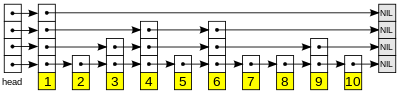​ 在前面我们介绍了有序数组中查找使用了二分法能够明显的降低时间复杂度，但是却无法降低插入的时间复杂（即使我们知道插入的位置在哪），这个是由数组的特性决定的（假如插入的值在数组的中间，后面的数据就不得不进行移动位置）。

​ 而在链表中，即使我们却不得不进行遍历，才能查找到结点。而链表的插入的时间却又是很快的（它并不需要 进行移动位置）。

​ 跳跃链表刚好集成了两者的优点，让我们能够更好的查找元素和插入元素。

• 每一个结点有两个指针，一个指向下一个结点，一个指向下一层结点。
• 如果一个第i层包含A结点，那么比i小的层都包含A结点（这一点从上面的图中可以含简单的看到）。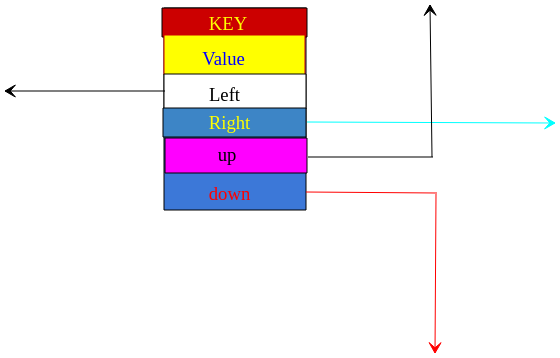Node模型

• 查找
• 插入
• 删除

public class SkipList<Key extends Comparable<Key>,Value>{

// 首先我们先定义好结点。
private class Node{
Key key;
Value value;
Node up;
Node down;
Node right;
Node left;

public Node(Key key, Value value){
this.key = key;
this.value = value;
}
}

// 当前跳表最大层数
private int maxLevel;
// 当前插入结点的个数
private int size;
// 首结点
// 尾结点
private Node tail;

public SkipList() {
maxLevel = 0;
// 创建首尾结点
tail = new Node(null,null);
size = 0;
}
}/**
* 通过key获得node(node不一定是正确的)
* @param key
* @return 返回的node.key <= key
*/
public Node getNode(Key key) {
if (key == null){
return  null;
}
while(true){
/**
* 假如node.right不为尾结点且key.value大于或者等于右边结点的key
*/
while (node.right.key != tail.key && key.compareTo(node.right.key) >= 0){
node = node.right;
}
if (node.down != null){
node = node.down;
}else {
break;
}
}
// 返回的node.key <= key
return node;
}

/**
* 通过key获得value
* @param key
* @return
*/
public Value get(Key key){
if (key == null){
return  null;
}

Node node = getNode(key);
if (node.key.compareTo(key) == 0){
return node.value;
}else {
return null;
}
}


1. 如果存在则修改。
2. 如果put的key值在跳跃链表中不存在，则进行新增节点，而高度由“天决定”。

/**
* 添加新的一层
*/
Node newTail= new Node(null,null);

newTail.down = tail;

tail.up = newTail;

tail = newTail;

maxLevel ++;
}

public void put(Key key, Value value) {
// Key不能为null
if (key == null){
return;
}
// 插入的合适位置
Node putPosition = getNode(key);
// 如果相等则更新
if (key.equals(putPosition.key)){
putPosition.value = value;
return;
}
// 进行新增操作
Node newNode = new Node(key,value);
/**
* putPostion的key小于key,所以排序位置应该是
* putPosition 【newNode】 putPosition.next
* 所以进行下面的操作
*/
newNode.right = putPosition.right;
newNode.left = putPosition;
putPosition.right.left = newNode;
putPosition.right = newNode;
Random random = new Random();
int level = 0;
// 产生0和1,使得新的结点有1/2的几率去增加level
while (random.nextInt(2) == 0){
// 假如高度达到了maxLevel则添加一个层
if (level >= maxLevel){
}
while (putPosition.up == null){
putPosition = putPosition.left;
}
putPosition = putPosition.up;
// 可以将skipNode中的value设置为空，不过为空也没什么关系
Node skipNode = new Node(key, null);
/**
* 需要的顺序是：
* putPosition 【skipNode】 putPosition.right
*/
skipNode.left = putPosition;
skipNode.right = putPosition.right;
putPosition.right.left = skipNode;
putPosition.right = skipNode;
// 将newNode放到上一层
/**
* putpostion skipNode skipNode.right
* newNode
*/
skipNode.down = newNode;
newNode.up = skipNode;
newNode = skipNode;
level ++;
}
size ++;
}

2层——>N*1/2，3层——>N*1/2*1/2，所以根据等比数列我们可以知道，除第1层以外所有的层数一共有N层

## 我家门前有几棵树

#### 树的介绍

• 每个节点都只有有限个子节点或无子节点；
• 没有父节点的节点称为根节点；
• 每一个非根节点有且只有一个父节点；
• 除了根节点外，每个子节点可以分为多个不相交的子树；
• 树里面没有环路(cycle)

#### 树的种类

• 无序树：树中任意节点的子节点之间没有顺序关系，这种树称为无序树，也称为自由树
• 有序树：树中任意节点的子节点之间有顺序关系，这种树称为有序树；
• 二叉树：每个节点最多含有两个子树的树称为二叉树；
• 完全二叉树：对于一颗二叉树，假设其深度为d（d>1）。除了第d层外，其它各层的节点数目均已达最大值，且第d层所有节点从左向右连续地紧密排列，这样的二叉树被称为完全二叉树；
• 满二叉树：所有叶节点都在最底层的完全二叉树；
• 平衡二叉树AVL树）：当且仅当任何节点的两棵子树的高度差不大于1的二叉树；
• 排序二叉树(二叉查找树（英语：Binary Search Tree))：也称二叉搜索树、有序二叉树；
• 霍夫曼树带权路径最短的二叉树称为哈夫曼树或最优二叉树；
• B树：一种对读写操作进行优化的自平衡的二叉查找树，能够保持数据有序，拥有多于两个子树。

### 二叉查找树(BST)1. 若某结点的左子树不为空，则左子树上面所有的节点的值都小于该节点。【8大于左边所有的值】
2. 若某结点的右子树不为空，则右子树上面所有的节点的值都大于该节点。【8小于右边所有的值】
3. 没有键相等的节点（也就是说所有节点的key都不相等）

public class BST<Key extends Comparable<Key>,Value> extends AbstractST <Key,Value> {

// 根节点
private Node root;

private class Node{
private Key key;
private Value value;
private Node left,right;
// 以该结点为根的子树中结点总数,包括该节点
private int N;

public Node(Key key, Value value, int n) {
this.key = key;
this.value = value;
this.N = n;
}
}

/**
* 查看是否含有key
* @param key
* @return
*/
public boolean containsNode(Key key){
if (key == null){
return false;
}
Node node = root;
int temp;
while (node!= null) {
temp = node.key.compareTo(key);
// 假如key小于结点的key,则转向左子树
if (temp > 0) {
node = node.left;
} else if (temp < 0) { // 转向右子树
node = node.right;
} else {
return true;
}
}
return false;
}
/**
* 获得查找二叉树中所有结点的数量
* @return
*/
@Override
public int size() {
return size(root);
}

/**
* 获得以某结点为根所有子树结点的数量（包括该结点）
* @param node
* @return
*/
public int size(Node node){
if (node == null){
return 0;
}
return node.N;
}
……省略一些了继承过来的方法
}

• 查找
@Override
public Value get(Key key) {
// 默认返回值为空
Value resultValue = null;
// 假如key为空或则二叉树为空
if (key == null){
return resultValue;
}

Node node = root;
int temp;
// 可以使用递归或者非递归实现
while (node!= null && (temp=node.key.compareTo(key)) != 0){
// 假如key小于结点的key,则转向左子树
if (temp>0){
node = node.left;
}else if (temp < 0){ // 转向右子树
node = node.right;
}else {
resultValue = node.value;
break;
}
}
return resultValue;
}

• 插入

@Override
public void put(Key key, Value value) {
if(key == null){
return;
}
// 假如二叉树是空的
if (root == null){
root = new Node(key,value,1);
return;
}
// 假如树中含有key,则进行更新，所以树中的N就不需要发生变化。
if (containsNode(key)){
}
int temp;
Node node = root;
while(true){
temp = node.key.compareTo(key);
// key相等则进行更新
if (temp == 0){
node.value = value;
return;
}
// 插入的key比node的key小，转向左子树
if (temp>0){
if (node.left == null){
node.left = new Node(key,value,1);
}
node = node.left;
}else {
if (node.right == null){
node.right = new Node(key,value,1);
}
node = node.right;
}
}

}
• 删除

删除应该可以说是树中最难的一个操作了。在查找二叉树中，删除分为一下3种情况：

1. 删除的结点是一个叶子结点，也就是说结点没有子节点。这种情况最容易处理。图源【在这位大佬的博客中，很生动形象的讲了删除的操作】。在这种情况下，我们可以直接将图中的结点7.right = null即可。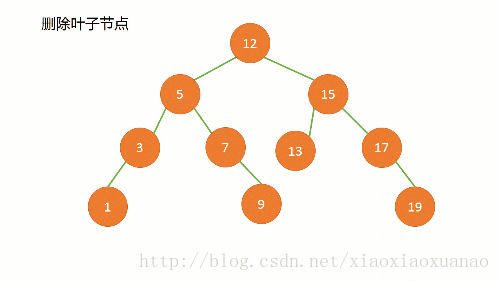删除叶子结点

2. 要删除的结点有一个子节点。这种情况也不复杂，如图所示的操作即可，同时被删除的结点的子节点上升了一个层次。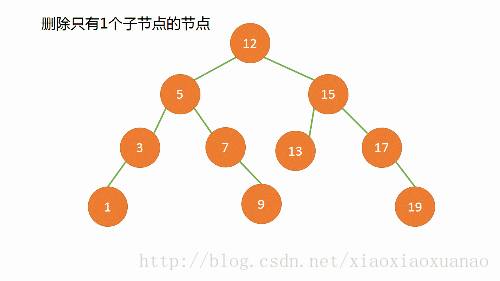有一个子节点

3. 要删除的结点有两个子节点，这种情况是最复杂的。因为父节点的left或者right无法同时指向被删除结点的两个子节点。解决这这个问题的方案有两种方案：合并删除和复制删除。

合并删除

首先先说一下合并删除，先给搭建看一看来自geeksforgeeks的一张图（稍微修改了一下）。

为什么叫合并删除呢？因为合并删除的思想就是将被删除节点的两个子节点合并成一棵树，然后代替被删除结点的位置，下面我将根据下图来讲一下具体的操作。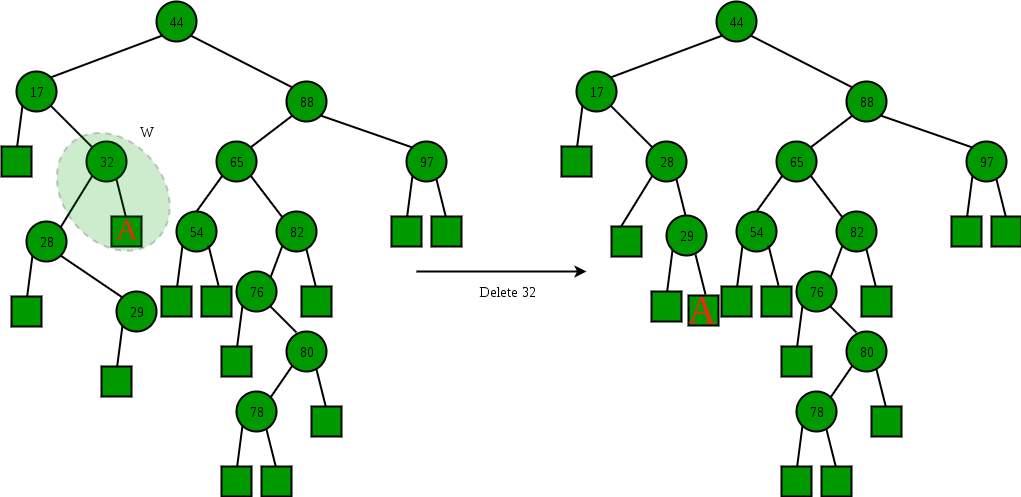合并删除

在图中我们需要删除的结点是32

1. 首先我们在删除结点的左子树找到最右边的结点Ｘ（也就是图中是结点29）。
2. 然后将结点Ｘ的右子节点指向被删除结点的右子节点。（也就是将29结点的右子节点指向结点Ａ）。
3. 最后使得被删除结点的父节点指向被删除结点的左子结点。（也就是17结点指向28结点）。

我们可以想一想为什么要这么做？根据二叉查找树的性质我们可以很简单的知道，Ａ子树是一定大于被删除结点的做子树的，所以将Ａ子树放在左子树的最右边。

首先，我们让我们来看看delete函数。

/**
* 进行删除
* @param key
* @return 返回删除结点的value
*/
@Override
public Value delete(Key key){
// 如果不包含key
if (!containsNode(key)){
return null;
}
// preNode代表删除结点的父节点
Node node = root,preNode = root;
int temp;
while (true) {
temp = node.key.compareTo(key);
// 假如key小于结点的key,则转向左子树
if (temp > 0) {
preNode = node;
// 在删除的同时，将结点的N--
preNode.N --;
node = node.left;
} else if (temp < 0) { // 转向右子树
preNode.N --;
preNode = node;
node = node.right;
} else {
break;
}
}
// 被删除结点的返回值
Value value = node.value;

// mergeDelete代表合并删除
if (node == root){
root = mergeDelete(node);
}
// 假如删除的是父节点的左边的结点
else if (preNode.left!=null && preNode.left == node){
preNode.left = mergeDelete(node);
}else {
preNode.right = mergeDelete(node);
}
return value;
}

接下来是mergeDelete函数，代码的注释已经写的很清楚了，如果能够理解合并删除的原理，那么理解下面的代码也将轻而易举：

/**
* 使用合并删除
* @param node
* @return 返回的结点为已经进行删除后新的结点
*/
public Node mergeDelete(Node node){
// 假如没有右子树
if (node.right == null){
return node.left;
}
// 假如没有左子树
else if (node.left == null){
return node.right;
}
// 既有右子树也有左子树
else {
Node tempNode = node.left;
// 转向左子树中最右边的结点
while (tempNode.right != null){
tempNode= tempNode.right;
tempNode.N += size(node.right);
}
// 将删除结点的右子树放入正确的位置
tempNode.right = node.right;
node.left.N += size(node.right);
return node.left;
}
}

归并删除有一个很大的缺点，那就是删除结点会导致树的高度的增加。接下来让我们来看看复制删除是怎么解决这个问题的。

复制删除（拷贝删除）

在说复制删除之前，我们需要先熟悉二叉查找树的前驱和后继（根据中序遍历衍生出来的概念）。

• 前驱：A结点的前驱是其左子树中最右侧结点。
• 后继：A结点的后继是其右子树中最左侧结点。

那么复制删除的原理是什么样的呢？很简，使用删除结点的前驱或者后继代替被删除的结点即可。

以下图来讲一下复制删除的原理（图源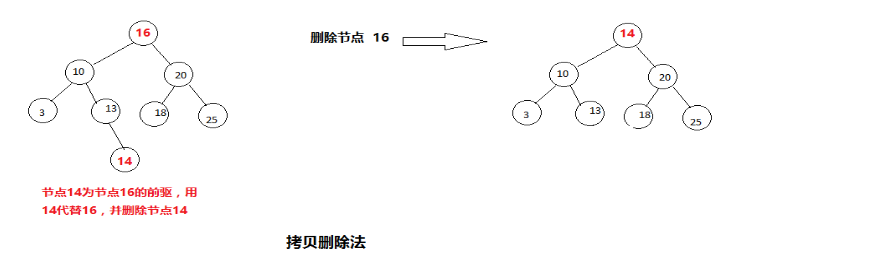16结点的前驱为14结点，后驱为18结点，假如我们要删除16结点，即可将14结点或者18结点替代16即可。

/**
* 使用复制删除
* @param node 被删除的结点
*/
private Node copyDelete(Node node){
if (node.right == null){
return node.left;
}else if(node.left == null){
return node.right;
}
// 既有左子树又有右子树
else {
Node tempNode = node.left;
while(tempNode.right != null){
tempNode.N --;
tempNode = tempNode.right;
}
tempNode.right = node.right;
tempNode.left = (node.left==tempNode?tempNode.left:node.left);
tempNode.N = size(tempNode.left) + size(tempNode.right)+1;
return tempNode;
}
}

// 调用删除函数
public Value deleteByCopy(Key key){
// 如果不包含key
if (!containsNode(key)){
return null;
}
Node node = root;
Node preNode = node;
int temp;
while (true){
node.N --;
temp = node.key.compareTo(key);
// 假如key小于结点的key,则转向左子树
if (temp > 0) {
preNode = node;
node = node.left;
} else if (temp < 0) { // 转向右子树
preNode = node;
node = node.right;
} else {
break;
}
}
// 被删除结点的返回值
Value value = node.value;
if (node == root){
root = copyDelete(node);
}
// 假如删除的是父节点的左边的结点
else if (preNode.left!=null && preNode.left == node){
preNode.left = copyDelete(node);
}else {
preNode.right = copyDelete(node);
}
return value;
}

J.Culberson从理论证实了使用非对称删除,IPL的期望值是O(n√n)，平均查找时间为（O(√n)），而使用对称删除，IPL的期望值为（nlgn）,平均查找时间为O(lgn)。

### 2-3 查找树

2–3树是一种树型数据结构，内部节点（存在子节点的节点）要么有2个孩子和1个数据元素，要么有3个孩子和2个数据元素，叶子节点没有孩子，并且有1个或2个数据元素。2个结点• 定义

如果一个内部节点拥有一个数据元素、两个子节点，则此节点为2节点

如果一个内部节点拥有两个数据元素、三个子节点，则此节点为3节点

当且仅当以下叙述中有一条成立时，T为2–3树：

• T为空。即T不包含任何节点。
• T为拥有数据元素a的2节点。若T的左孩子为L、右孩子为R，则
• LR是等高的非空2–3树；
• a大于L中的所有数据元素；
• a小于等于R中的所有数据元素。
• T为拥有数据元素ab的3节点，其中a < b。若T的左孩子为L、中孩子为M、右孩子为R，则
• LM、和R是等高的非空2–3树；
• a大于L中的所有数据元素，并且小于等于M中的所有数据元素；
• b大于M中的所有数据元素，并且小于等于R中的所有数据元素。

2-3查找树的查找和二叉树很类似，无非就是进行比较然后选择下一个查找的方向。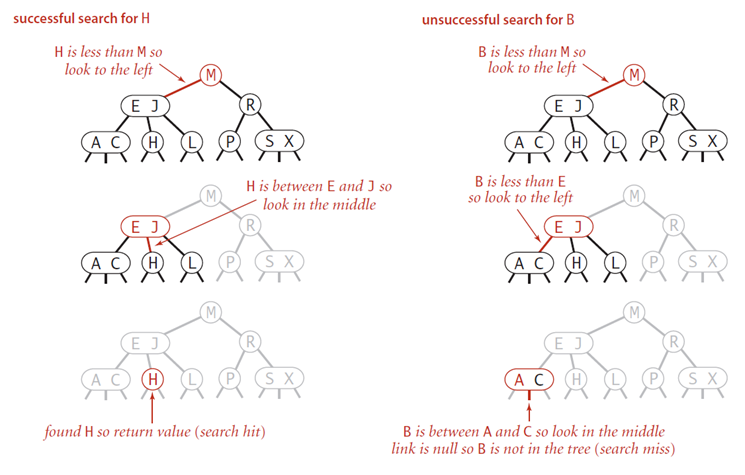2-3h奥

2-3a查找树的插入

**2-结点插入:**向2-结点插入一个新的结点和向而插入插入一个结点很类似，但是我们并不是将结点“吊”在结点的末尾，因为这样就没办法保持树的平衡。我们可以将2-结点替换成3-结点即可，将其中的键插入这个3-结点即可。（相当于缓存了这个结点）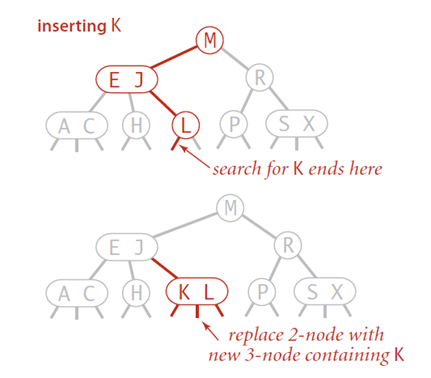**3-结点插入：**3结点插入比较麻烦，emm可以说是特别麻烦，它分为3种情况。

1. 向一棵只含有3-结点的树插入新键。

假如2-3树只有一个3-结点，那么当我们插入一个新的结点的时候，我们先假设结点变成了4-结点，然后使得中间的结点为根结点，左边的结点为其左结点，右边的结点为其右结点，然后构成一棵2-3树，树的高度加1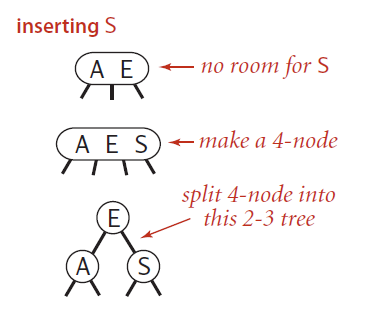2. 向父结点为2-结点的3-结点中插入新键。

和上面的情况类似，我们将新的节点插入3-结点使之成为4-结点，然后将结点中的中间结点”升“到其父节点（2-结点）中的合适的位置，使其父节点成为一个3-节点，然后将左右节点分别挂在这个3-结点的恰当位置，树的高度不发生改变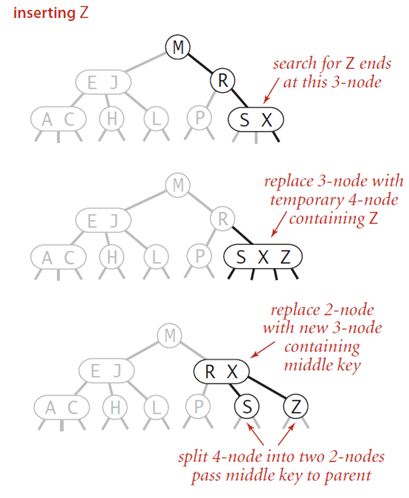3. 向父节点为3-结点的3-结点中插入新键。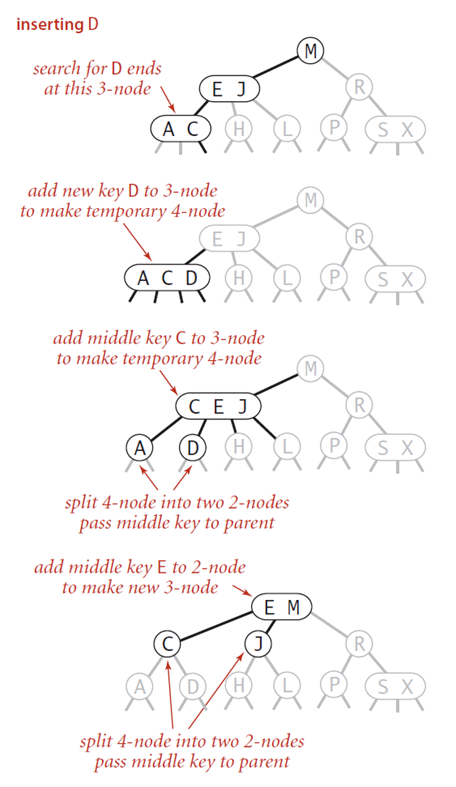public class Tree23<Key extends Comparable<Key>,Value> {
/**
* 保存key和value的键值对
* @param <Key>
* @param <Value>
*/
private class Data<Key extends Comparable<Key>,Value>{
private Key key;
private Value value;

public Data(Key key, Value value) {
this.key = key;
this.value = value;
}
public void displayData(){
System.out.println("/" + key+"---"+value);
}
}

/**
* 保存树结点的类
* @param <Key>
* @param <Value>
*/
private class Node23<Key extends Comparable<Key>,Value>{

public void displayNode() {
for(int i = 0; i < itemNum; i++){
itemDatas[i].displayData();
}
System.out.println("/");
}

private static final int N = 3;
// 该结点的父节点
private Node23 parent;
// 子节点，子节点有3个，分别是左子节点，中间子节点和右子节点
private Node23[] chirldNodes = new Node23[N];
// 代表结点保存的数据（为一个或者两个）
private Data[] itemDatas = new Data[N - 1];
// 结点保存的数据个数
private int itemNum = 0;

/**
* 判断是否是叶子结点
* @return
*/
private boolean isLeaf(){
// 假如不是叶子结点。必有左子树（可以想一想为什么？）
return chirldNodes == null;
}

/**
* 判断结点储存数据是否满了
* （也就是是否存了两个键值对）
* @return
*/
private boolean isFull(){
return itemNum == N-1;
}

/**
* 返回该节点的父节点
* @return
*/
private Node23 getParent(){
return this.parent;
}

/**
* 将子节点连接
* @param index 连接的位置（左子树，中子树，还是右子树）
* @param child
*/
private void connectChild(int index,Node23 child){
chirldNodes[index] = child;
if (child != null){
child.parent = this;
}
}

/**
* 解除该节点和某个结点之间的连接
* @param index 解除链接的位置
* @return
*/
private Node23 disconnectChild(int index){
Node23 temp = chirldNodes[index];
chirldNodes[index] = null;
return temp;
}

/**
* 获取结点左或右的键值对
* @param index 0为左，1为右
* @return
*/
private Data getData(int index){
return itemDatas[index];
}

/**
* 获得某个位置的子树
* @param index 0为左指数，1为中子树，2为右子树
* @return
*/
private Node23 getChild(int index){
return chirldNodes[index];
}

/**
* @return 返回结点中键值对的数量，空则返回-1
*/
public int getItemNum(){
return itemNum;
}

/**
* 寻找key在结点的位置
* @param key
* @return 结点没有key则放回-1
*/
private int findItem(Key key){
for (int i = 0; i < itemNum; i++) {
if (itemDatas[i] == null){
break;
}else if (itemDatas[i].key.compareTo(key) == 0){
return i;
}
}
return -1;
}

/**
* 向结点插入键值对：前提是结点未满
* @param data
* @return 返回插入的位置 0或则1
*/
private int insertData(Data data){
itemNum ++;
for (int i = N -2; i >= 0 ; i--) {
if (itemDatas[i] == null){
continue;
}else{
if (data.key.compareTo(itemDatas[i].key)<0){
itemDatas[i+1] = itemDatas[i];
}else{
itemDatas[i+1] = data;
return i+1;
}
}
}
itemDatas = data;
return 0;
}

/**
* 移除最后一个键值对（也就是有右边的键值对则移右边的，没有则移左边的）
* @return 返回被移除的键值对
*/
private Data removeItem(){
Data temp = itemDatas[itemNum - 1];
itemDatas[itemNum - 1] = null;
itemNum --;
return temp;
}
}
/**
* 根节点
*/
private Node23 root = new Node23();
……接下来就是一堆方法了
}

/**
*查找含有key的键值对
* @param key
* @return 返回键值对中的value
*/
public Value find(Key key) {
Node23 curNode = root;
int childNum;
while (true) {
if ((childNum = curNode.findItem(key)) != -1) {
return (Value) curNode.itemDatas[childNum].value;
}
// 假如到了叶子节点还没有找到，则树中不包含key
else if (curNode.isLeaf()) {
return null;
} else {
curNode = getNextChild(curNode,key);
}
}
}

/**
* 在key的条件下获得结点的子节点（可能为左子结点，中间子节点，右子节点）
* @param node
* @param key
* @return 返回子节点，若结点包含key,则返回传参结点
*/
private Node23 getNextChild(Node23 node,Key key){
for (int i = 0; i < node.getItemNum(); i++) {
if (node.getData(i).key.compareTo(key)>0){
return node.getChild(i);
}
else if (node.getData(i).key.compareTo(key) == 0){
return node;
}
}
return node.getChild(node.getItemNum());
}

/**
* 最重要的插入函数
* @param key
* @param value
*/
public void insert(Key key,Value value){
Data data = new Data(key,value);
Node23 curNode = root;
// 一直找到叶节点
while(true){
if (curNode.isLeaf()){
break;
}else{
curNode = getNextChild(curNode,key);
for (int i = 0; i < curNode.getItemNum(); i++) {
// 假如key在node中则进行更新
if (curNode.getData(i).key.compareTo(key) == 0){
curNode.getData(i).value =value;
return;
}
}
}
}

// 若插入key的结点已经满了，即3-结点插入
if (curNode.isFull()){
split(curNode,data);
}
// 2-结点插入
else {
// 直接插入即可
curNode.insertData(data);
}
}

/**
* 这个函数是裂变函数，主要是裂变结点。
* 这个函数有点复杂，我们要把握住原理就好了
* @param node 被裂变的结点
* @param data 要被保存的键值对
*/
private void split(Node23 node, Data data) {
Node23 parent = node.getParent();
// newNode用来保存最大的键值对
Node23 newNode = new Node23();
// newNode2用来保存中间key的键值对
Node23 newNode2 = new Node23();
Data mid;

if (data.key.compareTo(node.getData(0).key)<0){
newNode.insertData(node.removeItem());
mid = node.removeItem();
node.insertData(data);
}else if (data.key.compareTo(node.getData(1).key)<0){
newNode.insertData(node.removeItem());
mid = data;
}else{
mid = node.removeItem();
newNode.insertData(data);
}
if (node == root){
root = newNode2;
}
/**
* 将newNode2和node以及newNode连接起来
* 其中node连接到newNode2的左子树，newNode
* 连接到newNode2的右子树
*/
newNode2.insertData(mid);
newNode2.connectChild(0,node);
newNode2.connectChild(1,newNode);
/**
* 将结点的父节点和newNode2结点连接起来
*/
connectNode(parent,newNode2);
}

/**
* 链接node和parent
* @param parent
* @param node node中只含有一个键值对结点
*/
private void connectNode(Node23 parent, Node23 node) {
Data data = node.getData(0);
if (node == root){
return;
}
// 假如父节点为3-结点
if (parent.isFull()){
// 爷爷结点（爷爷救葫芦娃）
Node23 gParent = parent.getParent();
Node23 newNode = new Node23();
Node23 temp1,temp2;
Data itemData;

if (data.key.compareTo(parent.getData(0).key)<0){
temp1 = parent.disconnectChild(1);
temp2 = parent.disconnectChild(2);
newNode.connectChild(0,temp1);
newNode.connectChild(1,temp2);
newNode.insertData(parent.removeItem());

itemData = parent.removeItem();
parent.insertData(itemData);
parent.connectChild(0,node);
parent.connectChild(1,newNode);
}else if(data.key.compareTo(parent.getData(1).key)<0){
temp1 = parent.disconnectChild(0);
temp2 = parent.disconnectChild(2);
Node23 tempNode = new Node23();

newNode.insertData(parent.removeItem());
newNode.connectChild(0,newNode.disconnectChild(1));
newNode.connectChild(1,temp2);

tempNode.insertData(parent.removeItem());
tempNode.connectChild(0,temp1);
tempNode.connectChild(1,node.disconnectChild(0));

parent.insertData(node.removeItem());
parent.connectChild(0,tempNode);
parent.connectChild(1,newNode);
} else{
itemData = parent.removeItem();

newNode.insertData(parent.removeItem());
newNode.connectChild(0,parent.disconnectChild(0));
newNode.connectChild(1,parent.disconnectChild(1));
parent.disconnectChild(2);
parent.insertData(itemData);
parent.connectChild(0,newNode);
parent.connectChild(1,node);
}
// 进行递归
connectNode(gParent,parent);
}
// 假如父节点为2结点
else{
if (data.key.compareTo(parent.getData(0).key)<0){
Node23 tempNode = parent.disconnectChild(1);
parent.connectChild(0,node.disconnectChild(0));
parent.connectChild(1,node.disconnectChild(1));
parent.connectChild(2,tempNode);
}else{
parent.connectChild(1,node.disconnectChild(0));
parent.connectChild(2,node.disconnectChild(1));
}
parent.insertData(node.getData(0));
}
}


2-3树的查找效率与树的高度相关，这个可以很简单的理解，因为2-3树是平衡树，所以即使是最坏的情况下，它然仍是一棵树，不会产生类似链表的情况。

• 最坏情况：全是2-结点，树的高度最大，查找效率为lgN。
• 最好情况：全是3-结点，树的高度最小，查找效率为log3(N)，约等于0.631lgN。

2-3查找树的原理很简单，甚至说代码实现起来难度都不是很大，但是却很繁琐，因为它有很多种情况，结束完欲仙欲死的2-3查找树，接下来让我们来好好的研究一下红黑树。

### 红黑树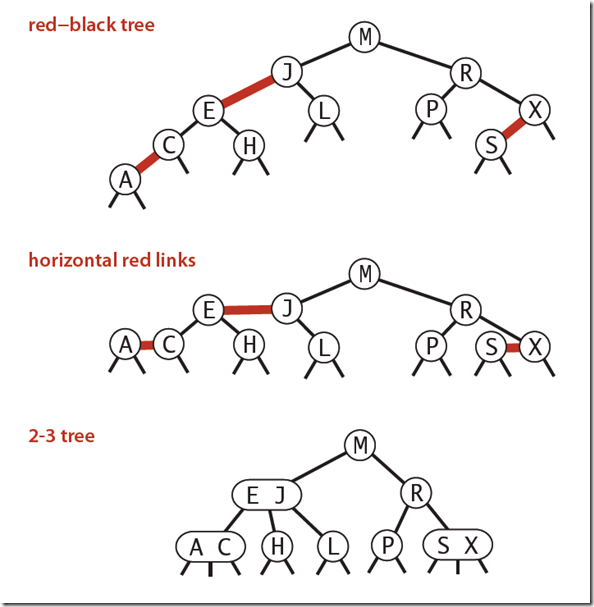• 红链接将两个2-结点链接起来构成了一个3-结点。
• 黑链接则是一个2-3树中普通的链接。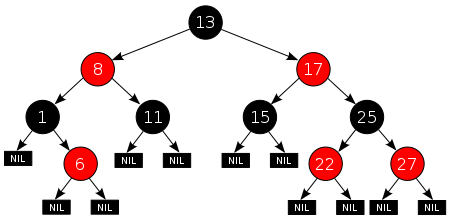(图源)

1. 节点是红色或黑色。
2. 根是黑色。
3. 所有叶子结点都是黑色（叶子是NIL节点）。
4. 每个红色节点必须有两个黑色的子节点。（从每个叶子到根的所有路径上不能有两个连续的红色节点。）
5. 从任一节点到其每个叶子的所有简单路径都包含相同数目的黑色节点。

• 从根到叶子的最长距离不会大于最短距离的两倍长。（由第4条和第5条性质保证）这样就保证了树的平衡性。

public class RBTree<Key extends Comparable<Key>,Value> {
private static final boolean RED = true;
private static final boolean BLACK = false;
private class Node<Key extends Comparable<Key>,Value>{
private Key key;
private Value value;
private boolean color;
private Node rightNode,leftNode;
// 这棵子树中结点的个数，包括该节点
private int N;
private Node root;
public Node(Key key, Value value, boolean color, int n) {
this.key = key;
this.value = value;
this.color = color;
N = n;
}
}

/**
* 获得改结点与其父节点之间链接的颜色
* @param node
* @return
*/
private boolean isRed(Node node){
if (node == null){
return false;
}
return node.color;
}
……其他方法

}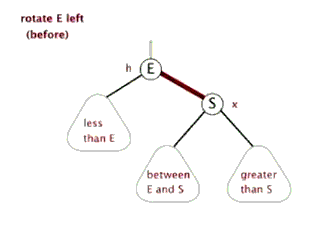/**
* 左旋转
* @param h
* @return 返回根节点
*/
private Node rotateLeft(Node h) {
Node x = h.rightNode;
h.rightNode = x.leftNode;
x.leftNode = h;
x.color = h.color;
h.color = RED;
x.N = h.N;
h.N = size(h.leftNode)+size(h.rightNode)+1;
return x;
}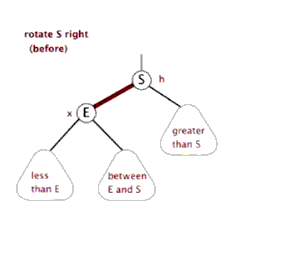/**
* 右旋转
* @param h
* @return 返回根节点
*/
private Node rotateRight(Node h) {
Node x = h.leftNode;
h.leftNode = x.rightNode;
x.rightNode = h;
x.color = h.color;
h.color = RED;
x.N = h.N;
h.N = size(h.leftNode)+size(h.rightNode)+1;
return x;
}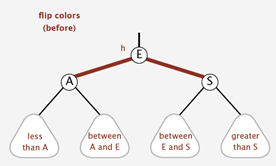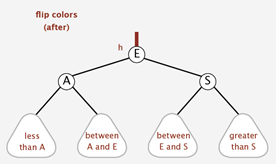/**
* 颜色转换
* @param h
*/
private void changeColor(Node h){
h.color = !h.color;
h.leftNode.color = !h.leftNode.color;
h.rightNode.color = h.rightNode.color;
}

2-结点插入：2-结点插入实际上就是其父节点没有红链接与之相连接

• 根结点插入（向左插入和向右插入）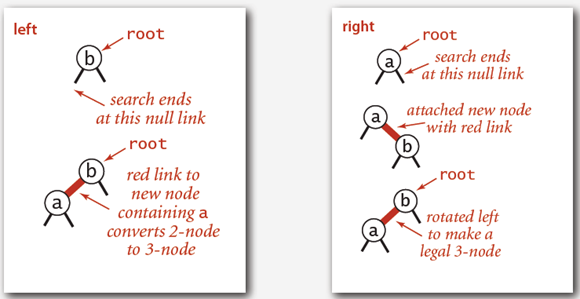• 叶子结点插入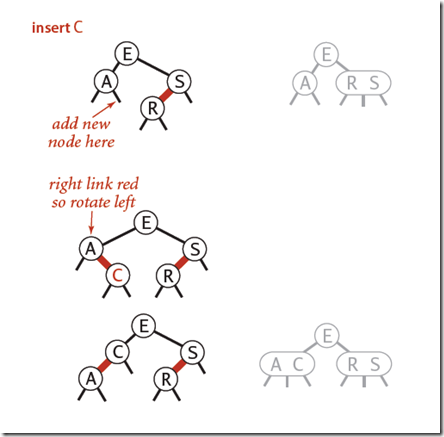**3-结点插入：**也就是其父节点有一个红链接与之相连接。

• 一个3-结点插入

在下图中，分为了三种情况，larger（新键最大），smaller（新键最小），between（新键介于两者中间）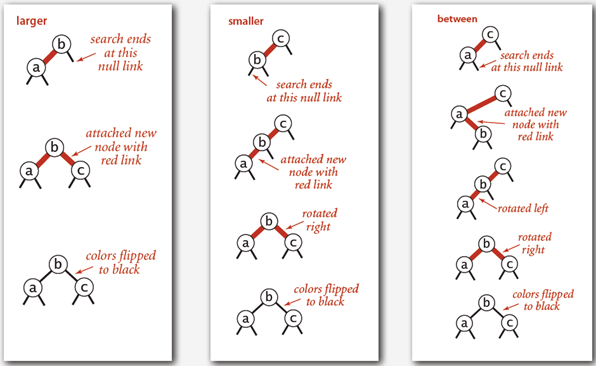• 向树底部插入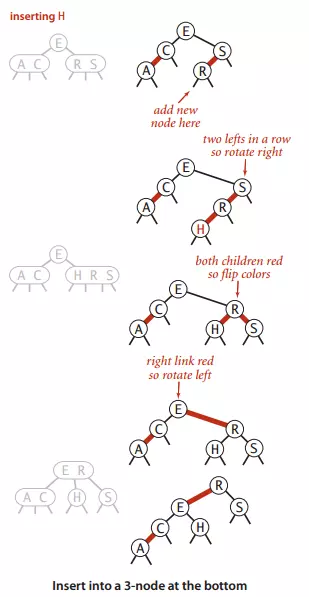• 若右子节点为红色而左子节点为黑色，则左旋转
• 若左子节点为红色且左子节点的左子节点也为红色则右旋转
• 左右结点都为红色，则颜色转换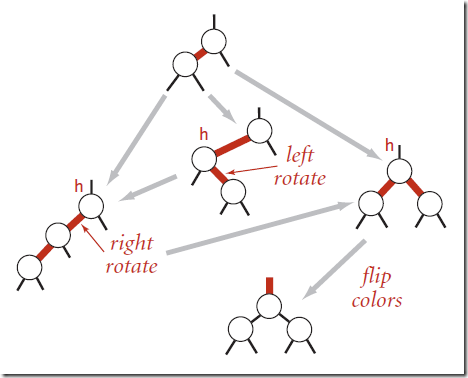/**
* 红黑树的插入
* @param key
* @param value
*/
public void put(Key key,Value value){
if(key == null){
return ;
}
root = put(root,key,value);
// 进行颜色变换会将本身结点变红，而根节点必须保持黑色
root.color = BLACK;
}

/**
* 插入操作进行递归调用
* @param h
* @param key
* @param value
* @return
*/
private Node put(Node h, Key key, Value value) {
// 当h为叶子结点的左子树或者右子树
if (h == null){
return new Node(key,value,RED,1);
}
// 假如key小于结点的key,就转向左结点
if (key.compareTo(h.key)<0){
h.leftNode = put(h.leftNode,key,value);
}
// 转向右结点
else if (key.compareTo(h.key)>0){
h.rightNode = put(h.rightNode,key,value);
}
// 更新
else{
h.value = value;
}
// 若左边结点是黑色，右边结点是红色，则左旋转
if (isRed(h.rightNode) && !isRed(h.leftNode)){
h = rotateLeft(h);
}
// 若左子节点为红色且左子节点的左子节点也为红色则右旋转
if (isRed(h.leftNode) && isRed(h.leftNode.leftNode)){
h = rotateRight(h);
}
// 左右结点都为红色，则颜色转换
if (isRed(h.leftNode) && isRed(h.rightNode)){
changeColor(h);
}
h.N = size(h.leftNode)+size(h.rightNode) + 1;
return h;
}

• 删除红色叶子结点
• 删除黑色叶子结点

​ 删除红色的叶子结点并没有什么好说的，直接删除，因为删除红色的叶子结点并不会影响破坏红黑树的平衡。因为我们知道一个红色结点绝对不可能存在只有左结点或者只有右结点的情况（可以解释下：若存在左子结点或右子结点，则子节点绝对会是黑色的，那么则会违反“从任一节点到其每个叶子的所有简单路径都包含相同数目的黑色节点”这个性质）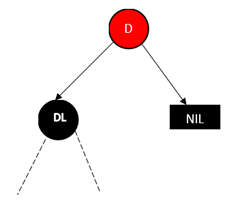• 删除的黑色结点只有左子结点或者右子结点
• 删除的黑色结点没有子结点
1. 删除的黑色结点只有左子结点或者右子结点

前面我们知道，我们使用的是前驱或者后继结点来替代被删除的结点，那么被删除的结点只最多只有一个子节点。并且由于红黑树的性质我们知道情况只能为以下两种情况（可以想下为什么结点不能为黑色）：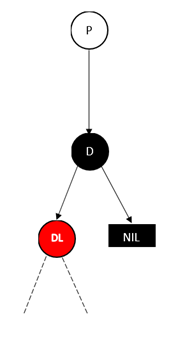前驱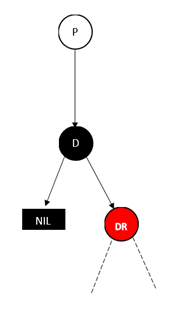对于这种，我们只需要将被删除黑结点的子节点替代被删除的结点即可，并将颜色改为black即可。

2. 删除的黑色结点没有子结点

这种情况是最复杂的，so，让我们来好好聊一聊这个东东。

2.1 待删除的节点的兄弟节点是红色的节点。

​ 因为删除的结点的兄弟结点是红色结点，我们进行左旋转，如图所示，我们可以发现红黑树的性质并没有被破害。

当然如果B结点在右边，我们使用右旋转就好了。这个时候情况就变成了下面2.2的那种情况。

图片来源美团技术团队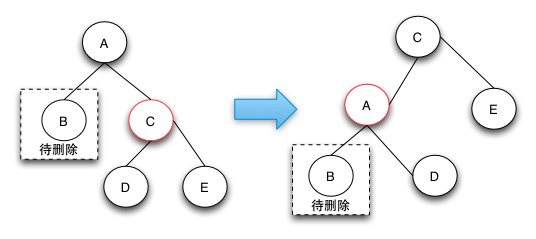2.2 待删除的节点的兄弟节点是黑色的节点，且兄弟节点的子节点都是黑色的。

下面的这幅图我纠结了很久，当时我看的时候，我一直在纠结为什么红黑树会出现下面的这种情况，因为它明显不符合红黑树的性质。我认为是博主搞错了，后来我又去看了圣书《算法导论》也是这样的，就更加的坚信是我想错了。

在这里我说下自己的理解：

在下面的图中，DE结点不一定是有数据的结点，也可能为NULL结点（NULL结点也是黑色的，这是我们的规定），如果为NULL结点，那么这种情况就回到了上图中的情况，B的兄弟结点D为黑色。 我们按照下图的操作，然后将D结点变成红色。在将B结点删除后，这个时候我们很容易的知道A结点的兄弟结点和A结点绝对不平衡了（违反了性质5），这个时候我们将B结点看成A结点以及它的子树C结点就看成A结点的兄弟结点。（这便是一个递归操作）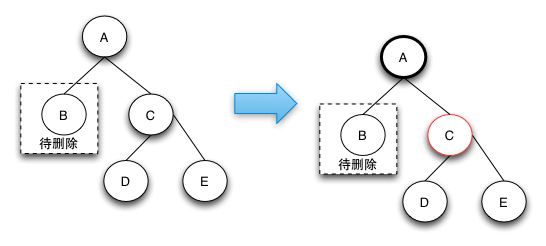2.3 待调整的节点的兄弟节点是黑色的节点，且兄弟节点的左子节点是红色的，右节点是黑色的(兄弟节点在右边)，如果兄弟节点在左边的话，就是兄弟节点的右子节点是红色的，左节点是黑色的。

这种状态我们可以理解为D是一棵左子树，E是一棵右子树，这样理解的话树还是可以保持平衡的。不过在*美团技术团队*上面说“他是通过case 2（也就是2.2操作）操作完后向上回溯出现的状态”，不过我没画出这幅图。

​ 在这种情况下我们可以这样理解：

在B子树中已经缺失了一个黑结点，那么我们必须要在D子树中和E子树中，让他们也损失一个黑结点（这个不像2.1我们直接将兄弟变红就行了，因为该节点的左结点为红结点），so，我们先将结点左旋转，得到2.4的情况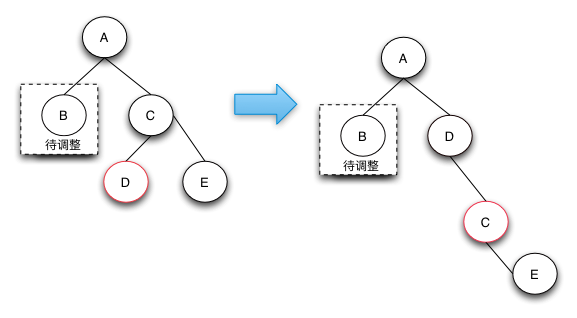2.4 待调整的节点的兄弟节点是黑色的节点，且右子节点是是红色的(兄弟节点在右边)，如果兄弟节点在左边，则就是对应的就是左节点是红色的。

​ 关于这个我们可以这样理解：

我们将D结点变红后，那边右边就要两个连续的红色结点了，so，我们需要左旋转，同时A结点变红。这样右边的结点就少了一个黑色的结点。树局部平衡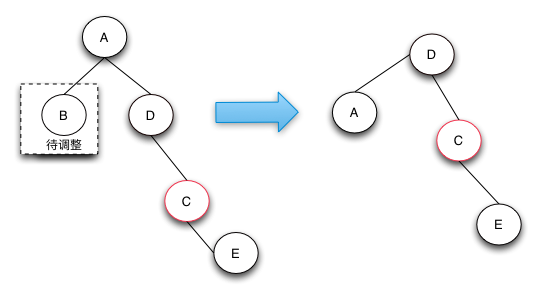• 若右子节点为红色而左子节点为黑色，则左旋转
• 若左子节点为红色且左子节点的左子节点也为红色则右旋转
• 左右结点都为红色，则颜色转换

/**
* 平衡树
* @param h
* @return
*/
private Node fixUp(Node h){
if (isRed(h.rightNode)){
h = rotateLeft(h);
}
if (isRed(h.leftNode) && isRed(h.leftNode.leftNode)){
h = rotateRight(h);
}
if (isRed(h.leftNode) && isRed(h.rightNode)){
changeColor(h);
}
h.N = size(h.leftNode)+size(h.rightNode)+1;
return h;
}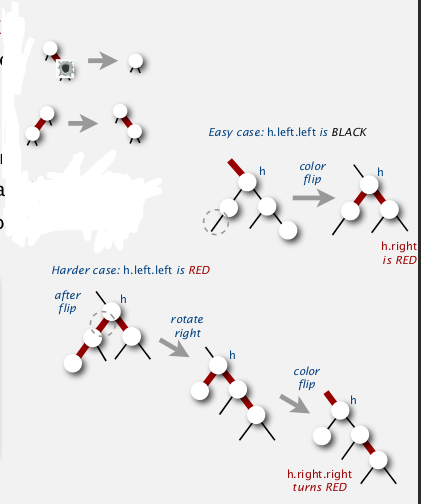/**
* 将红色结点移动到右边
* @param h
* @return
*/
private Node moveRedRight(Node h){
changeColor(h);
// 假如结点的左结点的左结点为红色结点
if (isRed(h.leftNode.leftNode)){
// 进行右转
h = rotateRight(h);
// 然后改颜色
changeColor(h);
}
return h;
}

public void deleteMax(){
if (root == null){
return;
}
if (!isRed(root.leftNode) && !isRed(root.rightNode)) {
root.color = RED;
}
root = deleteMax(root);
// 删除之后root不为空，则将root的颜色变为黑色
if (root != null){
root.color = BLACK;
}
}

private Node deleteMax(Node h) {
// 假如结点的左边是红色结点，则进行右旋转
if (isRed(h.leftNode)){
h = rotateRight(h);
}
// 如果右边为空的，则代表以及达到最大的结点
if (h.rightNode == null){
return null;
}
// 假如结点的右子节点为是黑色，右子节点的左子节点是黑色
// 在这种情况下，我们进行右旋转没办法得到将红色的结点转到右边来
// 所以我们执行moveRedRight并在里面创造红色的结点
if (!isRed(h.rightNode) && !isRed(h.rightNode.leftNode)){
h = moveRedRight(h);
}
h.rightNode = deleteMax(h.rightNode);
return fixUp(h);
}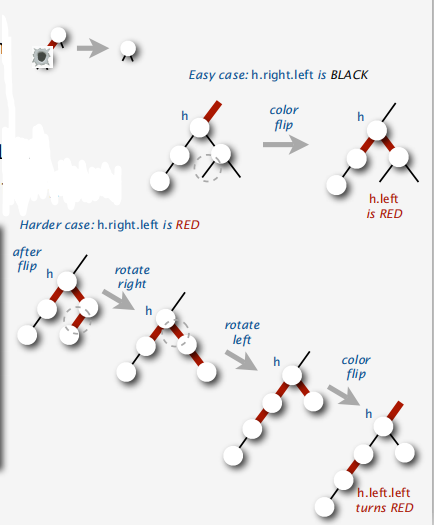/**
* 将红色结点移动到左边
* @param h
* @return
*/
private Node moveRedLeft(Node h){
changeColor(h);
if (isRed(h.rightNode.leftNode)){
h.rightNode = rotateLeft(h.rightNode);
h = rotateLeft(h);
changeColor(h);
}
return h;
}

public void deleteMin(){
if (root == null){
return;
}
if (!isRed(root.leftNode) && !isRed(root.rightNode)) {
root.color = RED;
}
root = deleteMin(root);
if (root != null){
root.color = BLACK;
}
}

private Node deleteMin(Node h) {
if (h.leftNode == null) {
return null;
}
if (!isRed(h.leftNode) && !isRed(h.leftNode.leftNode)){
h = moveRedLeft(h);
}
h.leftNode = deleteMin(h.leftNode);
return fixUp(h);
}

public void delete(Key key){
if (key == null){
return;
}
// 首先将结点变红以便于操作
if (!isRed(root.leftNode) && !isRed(root.rightNode)) {
root.color = RED;
}
root = delete(root,key);
if (root != null){
root.color = BLACK;
}

}

private Node delete(Node h, Key key) {
if (key.compareTo(h.key)<0){
if (!isRed(h.leftNode) && !isRed(h.leftNode.leftNode)){
h = moveRedLeft(h);
}
h.leftNode = delete(h.leftNode,key);
}
// 假如key比Node的key要大，或者相等
else{
// 左子节点为红色，则进行右旋转，这样能够将红色结点向右集中
if (isRed(h.leftNode)){
// 通过右转能够将红色结点上升
h = rotateRight(h);
}
// 这一步中，假如h的右结点为空，则h为叶子结点（此叶子结点并不代表NULL结点）
// 因为假如有左子节点的话，那么左子节点一定是红色（因为右子节点为空），那么在上面的一个if语句中h已经被右旋转到了右子节点
// 且h必定为红色的结点，这个时候我们就可以直接删除
if (key.compareTo(h.key) == 0 && h.rightNode == null){
return null;
}

if (!isRed(h.rightNode) && !isRed(h.rightNode.leftNode)){
h = moveRedRight(h);
}

if (key.compareTo(h.key) == 0){
// 找到h的后继结点，然后交换key和value，然后就可以删除最小节点了
Node x = min(h.rightNode);
h.key = x.key;
h.value = x.value;
h.rightNode = deleteMin(h.rightNode);
}
else{
h.rightNode = delete(h.rightNode,key);
}
}
return fixUp(h);
}
/**
* 找后继结点
* @param x
* @return
*/
private Node min(Node x) {
if (x.leftNode == null) {
return x;
}
else{
return min(x.leftNode);
}
}

## HASH大法好

### 散列表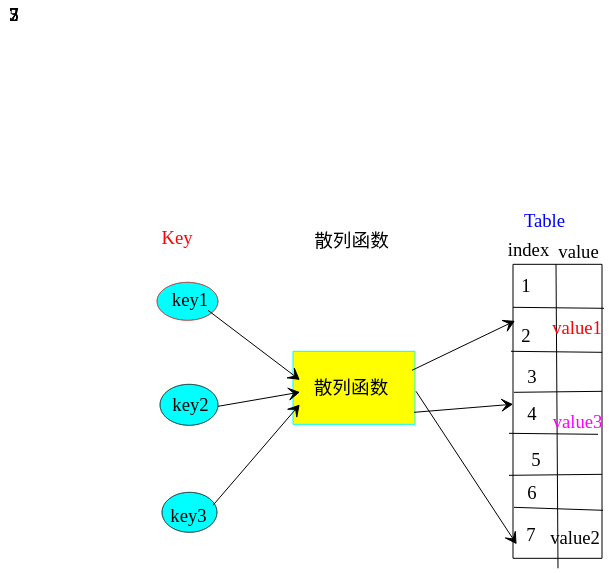• 一致性：等价的key必定要产生相等的散列值
• 高效性：计算简便
• 均匀性：均匀地散列所有的键

public class LinearHashST<Key extends Comparable<Key>,Value> {
private Key[] keys;
private Value[] values;
/**
* 键值对的数量
*/
private int N;

/**
* 默认数组大小
*/
private int M = 16;

public LinearHashST() {
keys = (Key[]) new Object[M];
values = (Value[]) new Object[M];
}

/**
* 初始化容量
* @param N 指令数组大小
*/
public LinearHashST(int N) {
M = N;
keys = (Key[]) new Object[M];
values = (Value[]) new Object[M];
}

private int hash(Key key){
return (key.hashCode()&0x7fffffff)%M;
}
public void put(Key key,Value value){
// 如果容量达到阀值，则扩容
if (N>=M*0.8){
resize(M*2);
}
// 得到hash值
int h;
for (h = hash(key);keys[h]!=null;h = (h+1)%M){
// key相等则更新
if (key.compareTo(keys[h]) == 0){
values[h] = value;
return;
}
}
keys[h] = key;
values[h] = value;
N ++;
}
public Value get(Key key){
int h;
for (h = hash(key);keys[h]!=null;h=(h+1)%M){
if (key.compareTo(keys[h]) == 0){
return values[h];
}
}
return null;
}
}

0123456789
A   S Z C D   F V G
A   NULL Z C D   F V G

/**
* 进行删除操作
* @param key
*/
public void delete(Key key){
int h = hash(key);
while(keys[h]!=null){
// 假如key存在
if (keys[h].compareTo(key) == 0){
keys[h] = null;
values[h] = null;
// 键值对数量减1
N--;
for (h=(h+1)%M; keys[h] != null;h=(h+1)%M){
// 将被删除结点后面的重新排列一下
Key keyToRedo = keys[h];
Value valToRedo = values[h];
keys[h] = null;
values[h] = null;
// 之所以N--是因为在put操作中N++了
N--;
put(keyToRedo,valToRedo);
}
// 缩小容量
if (N>0 && N == M/8){
resize(M/2);
}

}
h = (h+1)%M;
}
return;
}

/**
* 进行改变容量操作
* @param cap 容量大小
*/
private void resize(int cap){
LinearHashST<Key,Value> linearHashST = new LinearHashST(cap);
for (int i=0;i<M;i++) {
if (keys[i] != null){
linearHashST.put(keys[i],values[i]);
}
}
keys = linearHashST.keys;
values = linearHashST.values;
M = linearHashST.M;
}

​ 写完这篇博客我是一个头两个大，写的快哭了同时也快吐了，有史以来写的最吃力的一篇博客，其中在红黑树的删除花了自己将近3天的时间。谁让我这么菜呢，我能怎么办，我也很无奈啊。posted @ 2019-07-29 11:46  段小辉  阅读(1275)  评论(2编辑  收藏  举报